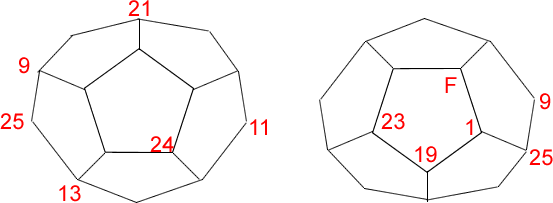#### You may also like### Prompt Cards

These two group activities use mathematical reasoning - one is numerical, one geometric.### Consecutive Numbers

An investigation involving adding and subtracting sets of consecutive numbers. Lots to find out, lots to explore.### Exploring Wild & Wonderful Number Patterns

EWWNP means Exploring Wild and Wonderful Number Patterns Created by Yourself! Investigate what happens if we create number patterns using some simple rules.

# Dodecamagic

##### Age 7 to 11Challenge LevelHere you see the front and back views of a dodecahedron which is a solid made up of pentagonal faces.

Using twenty of the numbers from $1$ to $25$, each vertex has been numbered so that the numbers around each pentagonal face add up to $65$.

The number F is the number of faces of the solid.

Can you find all the missing numbers?

You might like to make a dodecahedron and write the numbers at the vertices.